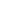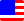Documents  >  Tutorial  >  Priority 7
Priority 7 operators

It describes the following operators with priority 7.

 == x == y Value equality operator != x != y Value inequality operator \$\$ x \$\$ y Reference equality operator !\$ x !\$ y Reference inequality operator

They are binary comparison operators. the equivalence of the left and right operands is compared and result is returned by the bool class.

There are two criteria for equivalence: value and reference.

Value equality operator

Value inequality operator

Reference equality operator

Reference inequality operator

Value equality operator

Value equality operator returns true when the left and right operands are equal in value. Otherwise it returns false.

bool result0 = 10 == 10; //The result is true;

bool result1 = 10 == 11; //The result is false;

bool result2 = "string" == "string"; //The result is true;

bool result3 = "string" == "strin"; //The result is false;

The operands of the value equality operator must be a class that represents the value. That is, int, long, real, string, bool classes.

When operands are string or bool class, the classes of the both operands must be the same. If the classes are different, an exception will be thrown.

When operands are int, long, or real class, You can mix these in the left and right operands.

In actuality, it is used as a conditional expression in if and while statements.

For example, if the variable i of the int class is defined, it is as follows.

if( i == 10 )

...

elseif( i == 11 )

...

endif

If operands return the proxy class, the entity of the proxy is automatically used. The result is the same as calling the Entity getter of the proxy class.

int i = 10;

real r = 10.5;

proxy pro = new proxy(i);

proxy pro2 = new proxy(r);

bool b = pro2 == pro; // b is false.

Value inequality operator

Value inequality operator returns true when the left and right operands are not equal in value. Otherwise it returns false.

bool result0 = 10 != 10; //The result is false;

bool result1 = 10 != 11; //The result is true;

bool result2 = "string" != "string"; //The result is false;

bool result3 = "string" != "strin"; //The result is true;

The operands of the value inequality operator must be a class that represents the value. That is, int, long, real, string, bool classes.

When operands are string or bool class, the classes of the both operands must be the same. If the classes are different, an exception will be thrown.

When operands are int, long, or real class, You can mix these in the left and right operands.

In actuality, it is used as a conditional expression in if and while statements.

For example, if the variable i of the int class is defined, it is as follows.

if( i != 10 )

...

elseif( i != 11 )

...

endif

If operands return the proxy class, the entity of the proxy is automatically used. The result is the same as calling the Entity getter of the proxy class.

int i = 10;

real r = 10.5;

proxy pro = new proxy(i);

proxy pro2 = new proxy(r);

bool b = pro2 != pro; // b is true.

Reference equality operator

Reference equality operator returns true when the left and right operands are equal as references. Otherwise it returns false.

int i = 10;

int j = i;

bool result0 = i \$\$ j; //The result is true;

bool result1 = i \$\$ 10; //The result is false;

There are no restrictions on the operands.

"Equal as a reference" means whether operandes are referring to the same address.

In actuality, it is used as a conditional expression in if and while statements.

For example, if the variable i and j of any class are defined, it is as follows.

if( i \$\$ j )

...

endif

Reference inequality operator

Reference inequality operator returns true when the left and right operands are not equal as references. Otherwise it returns false.

int i = 10;

int j = i;

bool result0 = i !\$ j; //The result is false;

bool result1 = i !\$ 10; //The result is true;

There are no restrictions on the operands.

"Equal as a reference" means whether operandes are referring to the same address.

In actuality, it is used as a conditional expression in if and while statements.

For example, if the variable i and j of any class are defined, it is as follows.

if( i !\$ j )

...

endif